# Galerie Fractale de Buttercup par L'intermédiaire de la Newton-RaphsonThumbnail Equation Interval Downloadable Resolution Comment Downloadf(x) = x3 - 1 Re[-3,3] Im[-3,3] 1200x1200 pixels 11 Iterations/pixel.  This was the first fractal that I constructed on Feb 11, 2004 Downloadf(x) = x3 - 1 Re[-.5,1] Im[0,1.5] 1200x1200 pixels 20 Iterations/pixel Downloadf(x)=x6+3 Re[.3916, 1.05833] Im[1.03351, 1.47795] 2700x1800 pixels 30 Iterations/pixel Downloadf(x) = x3+x2+x+1-sin(2x) Re[18Pi/5,23Pi/5] Im[2Pi/3, 4Pi/3] 2700x1800 pixels 30 Interations/pixel Downloadx = t - sin(t) y = 1 - cos(t) Re[17Pi/8, 25Pi/8] Im[-Pi/3, Pi/3] 3600x2400 pixels 20 Iterations/pixel Downloadx = r cos(t) y = r sin(t) Re[5Pi/32,13Pi/32] Im[-Pi/6, Pi/6] 3600x2700 pixels 20 Iterations/pixel Downloadf(x) = x5 - 1 Re[.5,1] Im[.3,.8] 1200x1200 pixels 2400x2400 pixels 25 Iterations/pixel Download MedRes Download HiResx = x2 + c Re[-2.1,.6] Im[-1.35,1.35] 1200x1200 pixels 2400x2400 pixels Mandelbrot.  Not Newton-Raphson Convergence.  100 Iterations/pixel DownloadMedRes Download HiResx = x2 + c Re[-.775,.-.725] Im[.05,.10] 600x600 pixels Hyper-detail of above fractal.  100 Iterations/pixel Downloadx = x2 + c Re[0.18, 0.50] Im[-0.62, -0.30] 2400x2400 pixels Hyper-detail of Mandelbrot fractal.  100 Iterations/pixel Download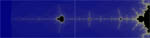x = x2 + c Re[-2.05, -1.34]   Im[-.08875, .08875] 2400x600 pixels Hyper-detail of Mandelbrot tail.  75 Iterations/pixel Downloadx = x2 + c Re[-2.1,.6] Im[-1.35,1.35] 1200x1200 pixels Mandelbrot.  Not Newton-Raphson Convergence.  100 Iterations/pixel Download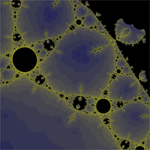f(x)=sin(1.25x)/x Re[2Pi,5Pi] Im[Pi,3Pi] 2700x1800 Pixels 25 Iterations/Pixel Downloadf(x) = x4 - 1 Re[1,2] Im[1,2] 1200x1200 pixels 15 Iterations/pixel Download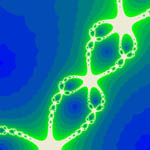f(x) = x3 - 1 Re[-.5,1] Im[0,1.5] 1200x1200 pixels 11 Iterations/Pixel.  Close up of the the first fractal I constructed. Downloadf(x) = x4 + x3 + 2x2 + .2x + 1 Re[-1.1. -.1] Im[1.9, 1.9] 1200x1200 Pixels 2400x2400 Pixels 30 Iterations/Pixel Download MedRes Download HiRes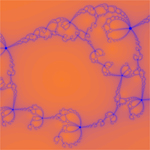f(x)=(x+1)5 Re[2,5] Im[2,4] 4500x3000 Pixels 25 Iterations/Pixel Downloadf(x)=exp(-x)sin(x) Re[-6Pi,0] Im[-2Pi,2Pi]  (Rotated) 1800x2700 Pixels 25 Iterations/Pixel Downloadf(x) = x8 + 2 Re[1.1775, 1.2875] Im[1.15, 1.26] 2880x1920 Pixels 100 Iterations/Pixel Download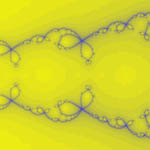f(x) = 2x4 + 3x3 + x2 + x + 1 Re[1.75. 3.25] Im[-.75, .75] 1200x1200 Pixels 2400x2400 Pixels 30 Iterations/Pixel Download MedRes Download HiResx = x2 + c Re[-2.6,-1.85 ] Im[-0.25, 0.25] 6480 x 1440 Pixels 40 Iterations/Pixel (in 3 Dimensions) Download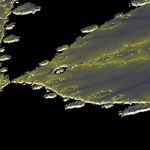f(x)=sin(1.25x)/x Re[2Pi,5Pi] Im[Pi,3Pi] 1650x508 Pixels 25 Iterations/Pixel (in 3 Dimensions) Downloadsf(x)=sin(x2) Re[-1.5,0] Im[-1,0] 1650x508 Pixels 25 Iterations/Pixel Downloads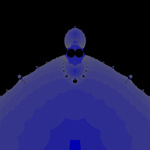f(x)=sin(1/x) Re[-1.5,1.5] Im[-1,1] 1619x974 Pixels 25 Iterations/Pixel Downloadsf(x)=x3sin(x) Re[-1,0] Im[-1/3,1/3] 1620x1216 Pixels 30 Iterations/Pixel Downloadsf(x)=e-xsin(x) Re[-3,3] Im[-2,2] 1619x1216 Pixels 25 Iterations/Pixel Downloads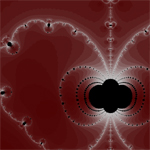f(x)=sin(x)/x2 Re[-1,1] Im[-2.5,-1.167] 1215x811 Pixels 25 Iterations/Pixel Downloadsf(x)=sin(x)/x2 Re[0,Pi] Im[0,2Pi/3] 1216x811 Pixels 25 Iterations/Pixel Downloads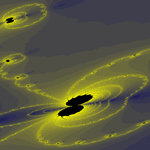f(x)=sin(x)ex Re[0.1,1.0] Im[0.45,1.05] 1024x544 Pixels 30 Iterations/Pixel Downloads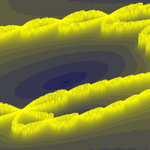f(x)=x3+1 Re[-0.15,1.35] Im[-0.53,0.47] 1920x1080 Pixels 20 Iterations/Pixel Downloadsf(x)=sin(x)ex Re[0,Pi/2] Im[-Pi/6,Pi/6] 3600x1231 Pixels 20 Iterations/Pixel Downloads